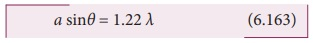Home | | Physics 12th Std | Resolution

# Resolution

The effect of diffraction has an adverse impact in the image formation by the optical instruments such as microscope and telescope.

Resolution

The effect of diffraction has an adverse impact in the image formation by the optical instruments such as microscope and telescope. For a single rectangular slit, the half angle ╬Ė subtended by the spread of central maximum (or position of first minimum) is given by the relation,

sin╬Ė ╬╗                 (6.162)Similar to a rectangular slit, when a circular aperture or opening (like a lens or the iris of our eye) forms an image of a point object, the image formed will not be a point but a diffraction pattern of concentric circles that becomes fainter while moving away from the center as shown in Figure 6.69. These are known as AiryŌĆÖs discs. The circle of central maximum has the half angular spread given by the equation,

a sin╬Ė = 1.22 ╬╗           (6.163)Here, the numerical value 1.22 comes for central maximum formed by circular apertures. This involves higher level mathematics which is avoided in this discussion.When two point sources close to each another form image on the screen, the diffraction pattern of one point source can overlap with another and produce a blurred image as shown in Figure 6.70(a). To obtain a good image of the two sources, the two point sources must be resolved i.e., the point sources must be imaged in such a way that their images are sufficiently far apart that their diffraction patterns do not overlap. According to RayleighŌĆÖs criterion, for two point objects to be just resolved, the minimum distance between their diffraction images must be in such a way that the central maximum of one coincides with the first minimum of the other and vice versa as shown in Figure 6.70(b). Such an image is said to be just resolved image of the object. The RayleighŌĆÖs criterion is said to be limit of resolution.According to RayleighŌĆÖs criterion the two point sources are said to be just resolved when the distance between the two maxima is at least ro. The angular resolution has a unit in radian (rad) and it is given by the equation,It shows that the first order diffraction angle must be as small as possible for greater resolution. This further shows that for better resolution, the wavelength of light used must be as small as possible and the size of the aperture of the instrument used must be as large as possible. The Equation 6.165 is used to calculate spacial resolution.

The inverse of resolution is called resolving power. This implies, smaller the resolution, greater is the resolving power of the instrument. The ability of an optical instrument to separate or distinguish small or closely adjacent objects through the image formation is said to be resolving power of the instrument. In general, the term resolution is pertaining to the quality of the image formed and the term resolving power is associated with the ability of the optical instrument.

### EXAMPLE 6.36

The optical telescope in the Vainu Bappu observatory at Kavalur has an objective lens of diameter 2.3 m. What is its angular resolution if the wavelength of light used is 589 nm?

### Solution

a = 2.3 m; ╬╗ = 589 nm = 589├Ś10-9 m; ╬Ė = ?

The equation for angular resolution is,

╬Ė = 1.22 ╬╗  a

Substituting,

╬Ė = 1.22├Ś589├Ś10ŌłÆ9 / 2.3 = 321.4├Ś10ŌłÆ9

╬Ė = 3.214├Ś10ŌłÆ7 rad Ōēł 0.0011'

Note: The angular resolution of human eye is approximately, 3├Ś10ŌłÆ4 rad Ōēł 1.03'.

Tags : Diffraction , 12th Physics : UNIT 7 : Wave Optics
Study Material, Lecturing Notes, Assignment, Reference, Wiki description explanation, brief detail
12th Physics : UNIT 7 : Wave Optics : Resolution | Diffraction